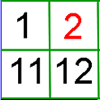#### You may also like### Prompt Cards

These two group activities use mathematical reasoning - one is numerical, one geometric.### Round and Round the Circle

What happens if you join every second point on this circle? How about every third point? Try with different steps and see if you can predict what will happen.### What's Left?

Use this grid to shade the numbers in the way described. Which numbers do you have left? Do you know what they are called?

# Dicey Array

##### Age 5 to 11Challenge Level

This is a game for two players.

You will need two 0-9 dice (or you could use our interactive dice), some counters, pen and paper.

Watch the video below (which has not got any sound). It shows two people playing the game.
Can you work out how to play?
What do you think the rules might be?

If you are unable to view the video, the rules of the game are hidden below.

The aim of the game:

To be the first player to reach the target score.

How to play:

1. Players agree a target score to reach.

2. Player 1 rolls the two dice and finds the total of the two numbers.

3. Player 1 counts out that number of counters and creates as many rectangular arrays as they can using that number of counters. They score a point for each correct array. (Each array must use all the counted-out counters.)

4. Player 2 rolls the dice in the same way and uses that number of counters to create as many rectangular arrays as possible. Again, they score a point for each correct array.

5. Play continues like this with players taking it in turns to roll the two dice. The points scored in each player's second turn are added to the number of points they scored in their first turn to make a running total. Points scored in their third turn are added on again, and so on.

6. The winner is the player who reaches the target number of points first.

Have a go at playing the game several times.

Which dice totals are good to get? Why?

Which dice totals are not so good to get? Why?

Does it matter if you go first or second?

What happens if you can choose to add or subtract the two dice numbers? How does this change the game?